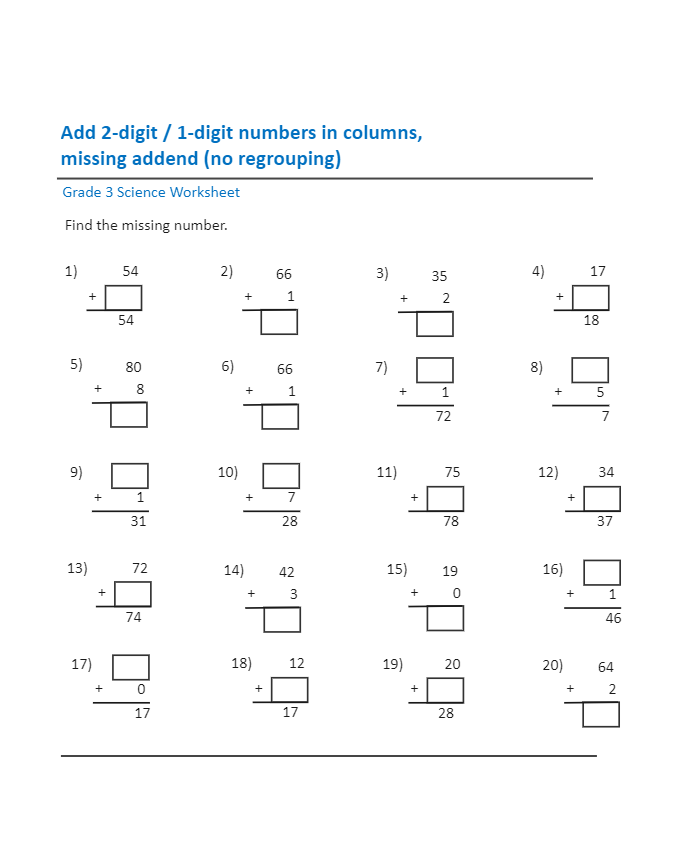# K-5 WorkesheetKiraaaa
Published on 2022-02-09
Edit OnlineFollowing K5 worksheet is dedicated to improving the basic mathematical skills of your students. Here the primary goal is to add digits in columns. As shown here, there are 20 mathematical equations where a particular digit(s) is missing. Your student's task is to fill up this worksheet and submit it to the teacher for valuation. Make sure that your mathematics worksheet contains a collection of similar math exercises. It would be easier for your students to get confused if different sets of questions were involved within one worksheet. With the help of EdrawMax, you can create K5 type of math worksheets that you can share with your students so even their parents can understand the work they have been doing in the classroom.
Tag
science diagram
Mathematics Diagram
Report
0
66PostRecommended Templates